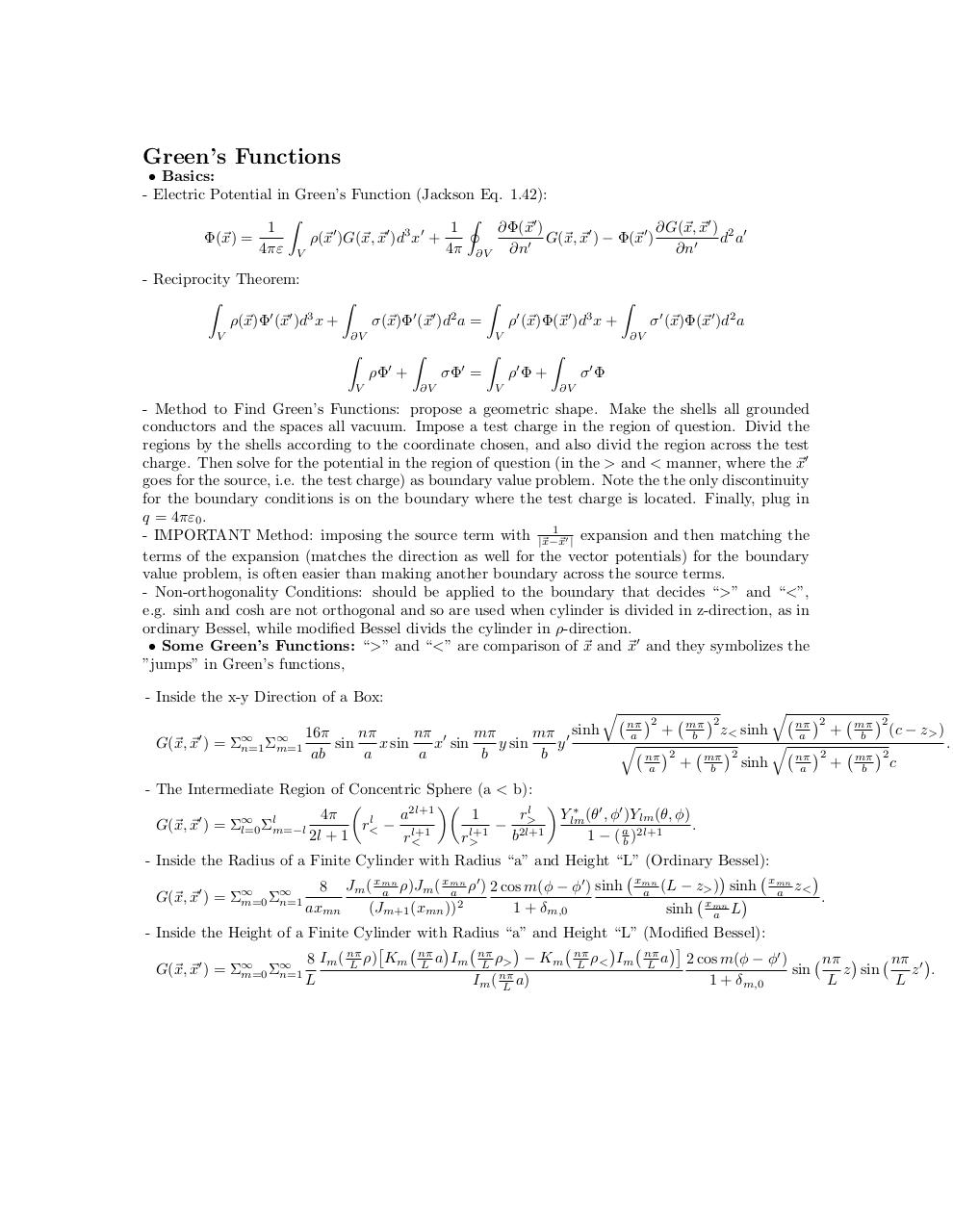# E&M.pdfPage 1 2 34515

#### Text preview

Green’s Functions
• Basics:
- Electric Potential in Green’s Function (Jackson Eq. 1.42):
Z
I
1
∂G(~x, ~x0 ) 2 0
1
∂Φ(~x0 )
Φ(~x) =
G(~x, ~x0 ) − Φ(~x0 )
d a
ρ(~x0 )G(~x, ~x0 )d3 x0 +
0
4πε V
4π ∂V ∂n
∂n0
- Reciprocity Theorem:
Z
Z
0 0 3
ρ(~x)Φ (~x )d x +
V

0

0

2

Z

σ(~x)Φ (~x )d a =

∂V

Z

0

0

Z

3

ρ (~x)Φ(~x )d x +
V

ρΦ0 +

V

Z
∂V

σΦ0 =

Z
V

σ 0 (~x)Φ(~x0 )d2 a

∂V

ρ0 Φ +

Z

σ0 Φ

∂V

- Method to Find Green’s Functions: propose a geometric shape. Make the shells all grounded
conductors and the spaces all vacuum. Impose a test charge in the region of question. Divid the
regions by the shells according to the coordinate chosen, and also divid the region across the test
charge. Then solve for the potential in the region of question (in the &gt; and &lt; manner, where the ~x0
goes for the source, i.e. the test charge) as boundary value problem. Note the the only discontinuity
for the boundary conditions is on the boundary where the test charge is located. Finally, plug in
q = 4πε0 .
1
- IMPORTANT Method: imposing the source term with |~x−~
x0 | expansion and then matching the
terms of the expansion (matches the direction as well for the vector potentials) for the boundary
value problem, is often easier than making another boundary across the source terms.
- Non-orthogonality Conditions: should be applied to the boundary that decides “&gt;” and “&lt;”,
e.g. sinh and cosh are not orthogonal and so are used when cylinder is divided in z-direction, as in
ordinary Bessel, while modified Bessel divids the cylinder in ρ-direction.
• Some Green’s Functions: “&gt;” and “&lt;” are comparison of ~x and ~x0 and they symbolizes the
”jumps” in Green’s functions,
- Inside the x-y Direction of a Box:

nπ 0

mπ 0 sinh
16π

sin
x sin
x sin
y sin
y
G(~x, ~x0 ) = Σ∞
n=1 Σm=1
ab
a
a
b
b

q


nπ 2
a

+
q

nπ 2
a


mπ 2
z&lt;
b
+


mπ 2
b

sinh
sinh

q


nπ 2
a

+


mπ 2
(c
b

q


nπ 2
a

+


mπ 2
c
b

− z&gt; )

- The Intermediate Region of Concentric Sphere (a &lt; b):


 ∗ 0 0
l
r&gt;

a2l+1
1
Ylm (θ , φ )Ylm (θ, φ)
0

l
l
G(~x, ~x ) = Σl=0 Σm=−l
r&lt; − l+1
− 2l+1
.
l+1
2l + 1
b
1 − ( ab )2l+1
r&lt;
r&gt;
- Inside the Radius of a Finite Cylinder with Radius “a” and Height “L” (Ordinary Bessel):


xmn 0
0 sinh xmn (L − z ) sinh xmn z
8 Jm ( xmn
&gt;
&lt;

a
a
a ρ)Jm ( a ρ ) 2 cos m(φ − φ )

G(~x, ~x0 ) = Σ∞
Σ
.
m=0 n=1
axmn
(Jm+1 (xmn ))2
1 + δm,0
sinh xmn
a L
- Inside the Height of a Finite Cylinder with Radius “a” and Height “L” (Modified Bessel):






2 cos m(φ − φ0 )
nπ 
nπ 0 
0

∞ 8 Im ( L ρ) Km L a Im L ρ&gt; − Km L ρ&lt; Im L a
G(~x, ~x ) = Σm=0 Σn=1
sin
z sin
z .

L
Im ( L a)
1 + δm,0
L
L

.